# Standard Deviation and Variance

Standard deviation and variance are statistical measures of dispersion of data, i.e., they represent how much variation there is from the average, or to what extent the values typically “deviate” from the mean (average). A variance or standard deviation of zero indicates that all the values are identical.

Variance is the mean of the squares of the deviations (i.e., difference in values from the mean), and the standard deviation is the square root of that variance. Standard deviation is used to identify outliers in the data.

## Important Concepts

• Mean: the average of all values in a data set (add all values and divide their sum by the number of values).
• Deviation: the distance of each value from the mean. If the mean is 3, a value of 5 has a deviation of 2 (subtract the mean from the value). Deviation can be positive or negative.

## Symbols

The formula for standard deviation and variance is often expressed using:

• x̅ = the mean, or average, of all data points in the problem
• X = an individual data point
• N = the number of points in the data set
• ∑ = the sum of [the squares of the deviations]

## Formulae

The variance of a set of n equally likely values can be written as: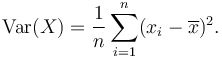$\operatorname{Var}(X) = \frac{1}{n} \sum_{i=1}^n (x_i - \overline{x})^2.$

The standard deviation is the square root of the variance: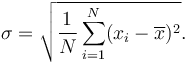$\sigma = \sqrt{\frac{1}{N} \sum_{i=1}^N (x_i - \overline{x})^2}.$

Formulae with Greek letters have a way of looking daunting, but this less complicated than it seems. To put it in simple steps:

1. find the average of all data points
2. find out how far each point is away from the average (this is the deviation)
3. square each deviation (i.e. the difference of each value from the mean)
4. divide the sum of the squares by the number of points.

That gives the variance. Take the square root of the variance to find the standard deviation.

## Example

Let’s say a data set includes the height of six dandelions: 3 inches, 4 inches, 5 inches, 4 inches, 11 inches, and 6 inches.

First, find the mean of the data points: (3 + 4 + 5 + 4 + 11 + 7) / 6 = 5.5

So the mean height is 5.5 inches. Now we need the deviations, so we find the difference of each plant from the mean: -2.5, -1.5, -.5, -1.5, 5.5, 1.5

Now square each deviation and find their sum: 6.25 + 2.25 + .25 + 2.25 + 30.25 + 2.25 = 43.5

Now divide the sum of the squares by the number of data points, in this case plants: 43.5 / 6 = 7.25

So the variance of this data set is 7.25, which is a fairly arbitrary number. To convert it into a real-world measurement, take the square root of 7.25 to find the standard deviation in inches.

The standard deviation is about 2.69 inches. That means that for the sample, any dandelion within 2.69 inches of the mean (5.5 inches) is ‘normal’.

### Why Square the Deviations?

Deviations are squared to prevent negative values (deviations below the mean) from canceling out the positive values. This works because a negative number squared becomes a positive value. If you had a simple data set with deviations from the mean of +5, +2, -1, and -6, the sum of the deviations will come out as zero if the values aren’t squared (i.e. 5 + 2 – 1 – 6 = 0).

## Real World Applications

Variance is expressed as a mathematical dispersion. Since it’s an arbitrary number relative to the original measurements of the data set, it is difficult to visualize and apply in a real-world sense. Finding the variance is usually just the final step before finding the standard deviation. Variance values are sometimes used in finance and statistical formulas.

Standard deviation, which is expressed in the original units of the data set, is much more intuitive and closer to the values of the original data set. It is most often used to analyze demographics or population samples to gain a sense of what is normal in the population.

### Finding outliers

In a normal distribution, about 68% of the population (or values) falls within 1 standard deviation (1σ) of the mean and about 94% fall within 2σ. Values that differ from the mean by 1.7σ or more are usually considered outliers.

In practice, quality systems like Six Sigma attempt to reduce the rate of errors so that errors become an outlier. The term “six sigma process” comes from the notion that if one has six standard deviations between the process mean and the nearest specification limit, practically no items will fail to meet specifications.

## Sample Standard Deviation

In real world applications, data sets used usually represent population samples, rather than entire populations. A slightly modified formula is used if population-wide conclusions are to be drawn from a partial sample.

A ‘sample standard deviation’ is used if all you have is a sample, but you wish to make a statement about the population standard deviation from which the sample is drawn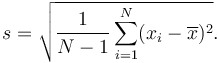$s = \sqrt{\frac{1}{N-1} \sum_{i=1}^N (x_i - \overline{x})^2}.$

The only way sample standard deviation formula differs from the standard deviation formula is the “-1” in the denominator.

Using the dandelion example, this formula would be needed if we sampled only 6 dandelions, but wanted to use that sample to state the standard deviation for the entire field with hundreds of dandelions.

The sum of squares would now be divided by 5 instead of 6 (n – 1), which gives a variance of 8.7 (instead of 7.25), and a sample standard deviation of 2.95 inches, instead of 2.69 inches for the original standard deviation. This change is used to find a margin of error in a sample (9% in this case).

Credits:

“Standard Deviation vs Variance.” Diffen.com. Diffen LLC, n.d. Web. 18 Apr 2016. < http://www.diffen.com/difference/Standard_Deviation_vs_Variance >

# Mean vs Median

## Definitions of mean and median

In mathematics and statistics, the mean or the arithmetic mean of a list of numbers is the sum of the entire list divided by the number of items in the list. When looking at symmetric distributions, the mean is probably the best measure to arrive at central tendency. In probability theory and statistics, a median is that number separating the higher half of a sample, a population, or a probability distribution, from the lower half.

## How to calculate

The Mean or average is probably the most commonly used method of describing central tendency. A mean is computed by adding up all the values and dividing that score by the number of values. The arithmetic mean of a sample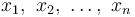$x_1,\ x_2,\ \ldots,\ x_n$ is the sum the sampled values divided by the number of items in the sample: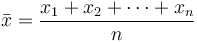$\bar{x} = \frac{x_1+x_2+\cdots +x_n}{n}$

The Median is the number found at the exact middle of the set of values. A median can be computed by listing all numbers in ascending order and then locating the number in the center of that distribution. This is applicable to an odd number list; in case of an even number of observations, there is no single middle value, so it is a usual practice to take the mean of the two middle values.

### Example

Let us say that there are nine students in a class with the following scores on a test: 2, 4, 5, 7, 8, 10, 12, 13, 83. In this case the average score (or the mean) is the sum of all the scores divided by nine. This works out to 144/9 = 16. Note that even though 16 is the arithmetic average, it is distorted by the unusually high score of 83 compared to other scores. Almost all of the students’ scores are below the average. Therefore, in this case the mean is not a good representative of the central tendency of this sample.

The median, on the other hand, is the value which is such that half the scores are above it and half the scores below. So in this example, the median is 8. There are four scores below and four above the value 8. So 8 represents the mid point or the central tendency of the sample.

Credits:”Mean vs Median.” Diffen.com. Diffen LLC, n.d. Web. 21 Apr 2016. < http://www.diffen.com/difference/Mean_vs_Median >